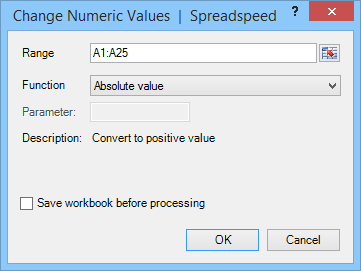Text Operations | Numeric Operations | Date Operations

# Excel Number Operations

Spreadspeed offers the following tools to perform numeric operations on a range of cells.

### Fill Range with Random Numbers

The Fill Random Values tool can fill a selected range with random numbers using either a uniform or normal distribution, with options to set the minimum value, maximum value, and the numbers of digits of precision. Note: The normal (Guassian) distribution values are generated using a Box-Muller algorithm.

##### Fill Random Values Screenshot### Change Numeric Values in Place Using Math Operations

This tool lets you perform a variety of mathemical operations on a selected range, updating the values in place.

Available Math Operations
Absolute value Floor Round
Acos Inverse Sin
Asin Log10 Sqrt
Atan Max Subtract
Ceiling Min Tan
Cos Modulo Tanh
Cosh Multiply Toggle Sign
Divide Negative Value Truncate
Exp Pow
##### Change Numeric Values Screenshot

The image below shows the Change Numeric Values dialog. After selecting a Function, the description field is updated to describe the operation and, if required, the Parameter field is enabled and re-labeled with the parameter name.### Other Numeric Operations

• Negate Selected Values: Inverts the sign of the selected values
• Convert Text to Numbers: Converts numbers stored as text into their numeric values. In addition to converting plain numbers, this tool also recognizes percentages (%), negative accounting formats, e.g. (5.00), and selected currency symbols (\$, €, £, ¥)
• Convert Formulas to Values: A quick alternative to using Copy and then Paste Special > Values.
• Round to 0 or 2 decimal places: Rounds the underlying value and sets number format to 0 or 2 decimal places

Text Operations | Numeric Operations | Date Operations